Courses

# RD Sharma Solutions -Ex-6.2, Fractions, Class 6, Maths Class 6 Notes | EduRev

## RD Sharma Solutions for Class 6 Mathematics

Created by: Abhishek Kapoor

## Class 6 : RD Sharma Solutions -Ex-6.2, Fractions, Class 6, Maths Class 6 Notes | EduRev

The document RD Sharma Solutions -Ex-6.2, Fractions, Class 6, Maths Class 6 Notes | EduRev is a part of the Class 6 Course RD Sharma Solutions for Class 6 Mathematics.
All you need of Class 6 at this link: Class 6

Q1. Represent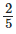on a number line.

Ans.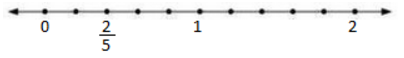Q2. Represent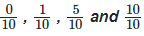on a number line.

Ans.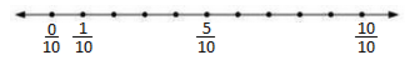Q3. Represent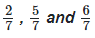on a number line.

Ans: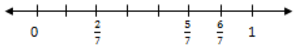Q4. How many fractions lie between 0 and 1?

Ans. Infinite. We can check this by taking numerator less than denominator in a fraction.

Q5. Represent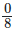and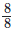on a number line.

Ans.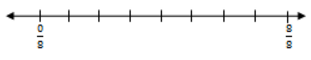103 docs

,

,

,

,

,

,

,

,

,

,

,

,

,

,

,

,

,

,

,

,

,

,

,

,

,

,

,

,

,

,

;